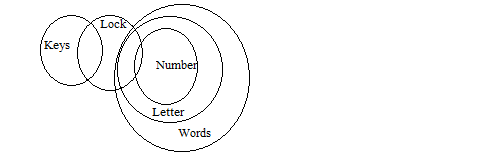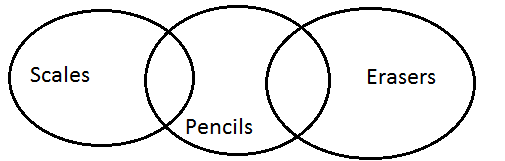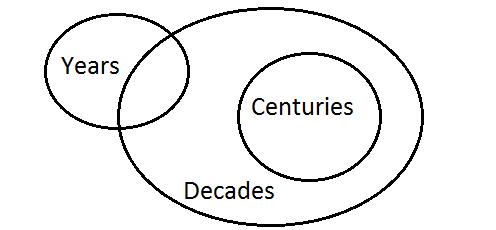home Home » Reasoning » Syllogims » Objective Questions

## Reasoning SyllogimsPage 3

###### Section-3
Direction:Two statements are given in the question, followed by two conclusions numbered 1 and 2. You have to take the given two statements to be true even, if they seem to be at variance from commonly known facts. Read the conclusions and then decide which of the given conclusions logically.

1.

 Statements: Some chairs are made up of wood. Some tables are made up of wood. Conclusions: 1. All wooden things are either chairs of tables. 2. Some chairs are tables. (a)If only Conclusion 1 follows (b)If only Conclusion 2 follows (c)If either Conclusion 1 or 2 follow (d)If neither Conclusion 1 nor 2 follow
Answer is: DFrom the above figure we cannot conclude that all wooden things are either chairs of tables and some chairs are tables. So none follows.

2.

 Statements: All crows are birds. All birds are peacocks. Conclusion: 1. All peacocks are birds. 2. All birds are peacocks. (a)If only Conclusion 1 follows (b)If only Conclusion 2 follows (c)If either Conclusion 1 or 2 follow (d)If neither Conclusion 1 nor 2 follow
Answer is: AFrom the above figure, it is clear that all peacocks are birds are not crows. Thus, only conclusion 1 follows.

3.

 Statements: Some keys are locks, some locks are numbers. All numbers are letters, all letters are words. Conclusions: 1. Some words are numbers. 2. Some locks are letters. (a)If only Conclusion 1 follows (b)If only Conclusion 2 follows (c)If either Conclusion 1 or 2 follow (d)If neither Conclusion 1 nor 2 follow
Answer is: CFrom, the above diagram, Some words are numbers and some locks are letters. So, Conclusions 1 and 2 follow.

4.

 Statements: Some scales are pencils. Some erasers are pencils. Conclusions: 1. Some pencils are erasers. 2. Some pencils are scales. (a)If only Conclusion 1 follows (b)If only Conclusion 2 follows (c)If either Conclusion 1 or 2 follow (d)If neither Conclusion 1 nor 2 follow
Answer is: CFrom the above figure, some pencils are erasers and some pencils are scales.

5.

 Statements: Some dews are drops. All drops are stones. Conclusions: 1. At-least some dews are stones. 2. At-least some stones are drops. (a)If only Conclusion 1 follows (b)If only Conclusion 2 follows (c)If either Conclusion 1 or 2 follow (d)If both Conclusions 1 and 2 follow
Answer is: DFrom the above figure, it is clear that at-least some dews are stones and at-least some stones are drops.

6.

 Statements: Some years are decades. All centuries are decades. Conclusions: 1. Some centuries are years. 2. Some decades are years. 3. No century is a year. (a)1 and 2 follow (b)1 and 3 follow (c)Either 1 or 3 and 2follow (d)None follows
Answer is: CFrom the above figure, it is clear that either 1 or 3 and 2 follows.

7.

 Statements: All philosophers are fools. All fools are illiterate. Conclusions: 1. All philosophers are illiterate. 2. All illiterate are philosopher. 3. All illiterate are fools. 4. Some illiterates would be philosopher. (a)Only 4 follow (b)1 and 4 follow (c)2 and 4 follw (d)3 and 4 follow
Answer is: BFrom the above figure, we can only conclude that all philosophers are illiterates some illiterates would be philosopher. So, only conclusions 1 and 4 follow.